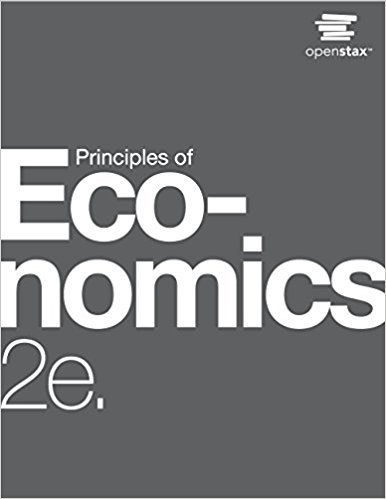×
Get Full Access to Principles Of Economics - 2 Edition - Chapter 17 - Problem 29
Get Full Access to Principles Of Economics - 2 Edition - Chapter 17 - Problem 29

×

# Solved: If you owned a small firm that had become somewhat established, but you needed aISBN: 9781947172364 471

## Solution for problem 29 Chapter 17

Principles of Economics | 2nd Edition

• Textbook Solutions
• 2901 Step-by-step solutions solved by professors and subject experts
• Get 24/7 help from StudySoup virtual teaching assistantsPrinciples of Economics | 2nd Edition

4 5 1 265 Reviews
25
5
Problem 29

If you owned a small firm that had become somewhat established, but you needed a surge of financial capital to carry out a major expansion, would you prefer to raise the funds through borrowing or by issuing stock? Explain your choice.

Step-by-Step Solution:
Step 1 of 3

Week 12 notes th March 30 Notes Monte Carol Simulation  Use when decisions are made with random events  Decision Tree: only random events with discrete outcomes o Discrete outcome=countable or limited number of outcomes  Monte Carlo Simulation: random events w/ discrete and continuous outcomes o Continuous outcome=every possible outcome in a range o Can use parametric and structural sensitivity easily  =RAND(x) function o Generates random number between 0 and 0.99999… o Re-calculates by pressing F9 (on PC) o Every number is equally likely (uniform distribution) o If make a histogram of 1000 trials of RAND fxn, all bars should be equal height  Uniform distribution ou

Step 2 of 3

Step 3 of 3

##### ISBN: 9781947172364

This textbook survival guide was created for the textbook: Principles of Economics, edition: 2. This full solution covers the following key subjects: . This expansive textbook survival guide covers 37 chapters, and 1291 solutions. The full step-by-step solution to problem: 29 from chapter: 17 was answered by , our top Business solution expert on 03/16/18, 04:24PM. The answer to “If you owned a small firm that had become somewhat established, but you needed a surge of financial capital to carry out a major expansion, would you prefer to raise the funds through borrowing or by issuing stock? Explain your choice.” is broken down into a number of easy to follow steps, and 41 words. Principles of Economics was written by and is associated to the ISBN: 9781947172364. Since the solution to 29 from 17 chapter was answered, more than 235 students have viewed the full step-by-step answer.

Unlock Textbook Solution